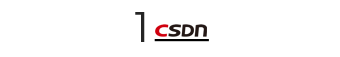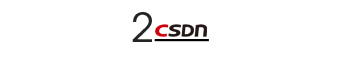``````from keras import layers
x = ...
y = layers.Conv2D(128, 3, activation='relu', padding='same')(x)
y = layers.Conv2D(128, 3, activation='relu', padding='same')(y)
y = layers.Conv2D(128, 3, activation='relu', padding='same')(y)

``````

``````from keras import layers

x = ... y = layers.Conv2D(128, 3, activation='relu', padding='same')(x) y = layers.Conv2D(128, 3, activation='relu', padding='same')(y) y = layers.MaxPooling2D(2, strides=2)(y)

residual = layers.Conv2D(128, 1, strides=2, padding='same')(x)

``````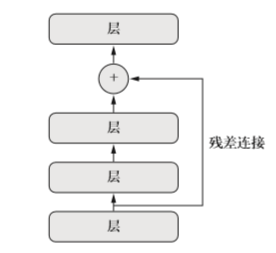``````normalized_data = (data - np.mean(data, axis=...)) / np.std(data, axis=...)
``````

• 批标准化的工作原理是，训练过程中在内部保存已读取每批数据均值 和方差的指数移动平均值。

• 批标准化的主要效果是，它有助于梯度传播（这一点和残差连接很 像），因此允许更深的网络。对于有些特别深的网络，只有包含多个 BatchNormalization 层 时才能进行训练。例如，BatchNormalization 广泛用于Keras 内置的许多高级卷积神经网络 架构，比如 ResNet50、Inception V3 和 Xception。

BatchNormalization 层通常在卷积层或密集连接层之后使用。

``````conv_model.add(layers.Conv2D(32, 3, activation='relu'))   conv_model.add(layers.BatchNormalization())

``````

BatchNormalization 层接收一个axis 参数，它指定应该对哪个特征轴做标准化。这 个参数的默认值是-1，即输入张量的最后一个轴。对于 Dense 层、Conv1D 层、RNN 层和将 data_format 设为 “channels_last”（通道在后）的 Conv2D 层，这个默认值都是正确的。但有少数人使用将 data_format 设为 “channels_first”（通道在前）的 Conv2D 层，这时 特征轴是编号为 1 的轴，因此 BatchNormalization 的 axis 参数应该相应地设为 1。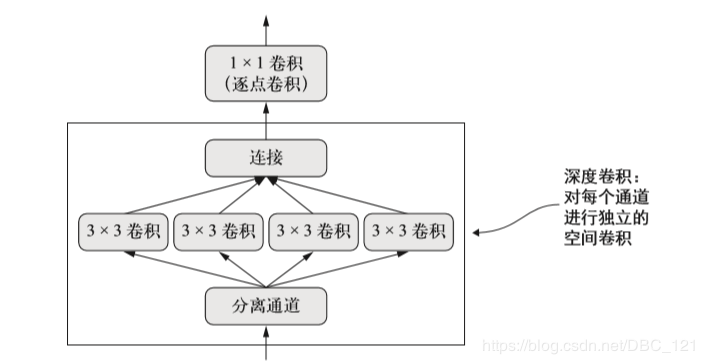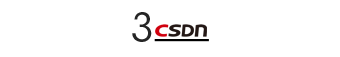• 选择一组超参数（自动选择）。

• 构建相应的模型。

• 将模型在训练数据上拟合，并衡量其在验证数据上的最终性能。

• 选择要尝试的下一组超参数（自动选择）。

• 重复上述过程。

• 最后，衡量模型在测试数据上的性能。

• 计算反馈信号（这组超参数在这个任务上是否得到了一个高性能的模型）的计算代价可能非常高，它需要在数据集上创建一个新模型并从头开始训练。

• 超参数空间通常由许多离散的决定组成，因而既不是连续的，也不是可微的。因此，你通常不能在超参数空间中做梯度下降。相反，你必须依赖不使用梯度的优化方法，而这些方法的效率比梯度下降要低很多。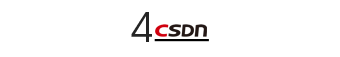``````preds_a = model_a.predict(x_val)
preds_b = model_b.predict(x_val)
preds_c = model_c.predict(x_val)
preds_d = model_d.predict(x_val)

final_preds = 0.25 * (preds_a + preds_b + preds_c + preds_d)
``````

``````preds_a = model_a.predict(x_val)
preds_b = model_b.predict(x_val)
preds_c = model_c.predict(x_val)
preds_d = model_d.predict(x_val)

final_preds = 0.5 * preds_a + 0.25 * preds_b + 0.1 * preds_c + 0.15 * preds_d
``````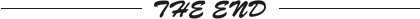``````更多精彩推荐
☞TensorFlow 2.0 发布以来，又有哪些最新进展？| AI ProCon 2020
☞疫情之下 SaaS 市场两极分化，SaaS 厂商如何突围严峻形势？
☞马斯克，带动大盘的男人：财报一出市值飙升 840 亿！
☞用 Python 可以实现侧脸转正脸？我也要试一下！
☞重大事故！线上系统频繁卡死，凶手竟然是 Full GC ？
☞以太坊第五年，未来该往哪走？

``````20年前，《新程序员》创刊时，我们的心愿是全面关注程序员成长，中国将拥有新一代世界级的程序员。20年后的今天，我们有了新的使命：助力中国IT技术人成长，成就一亿技术人！